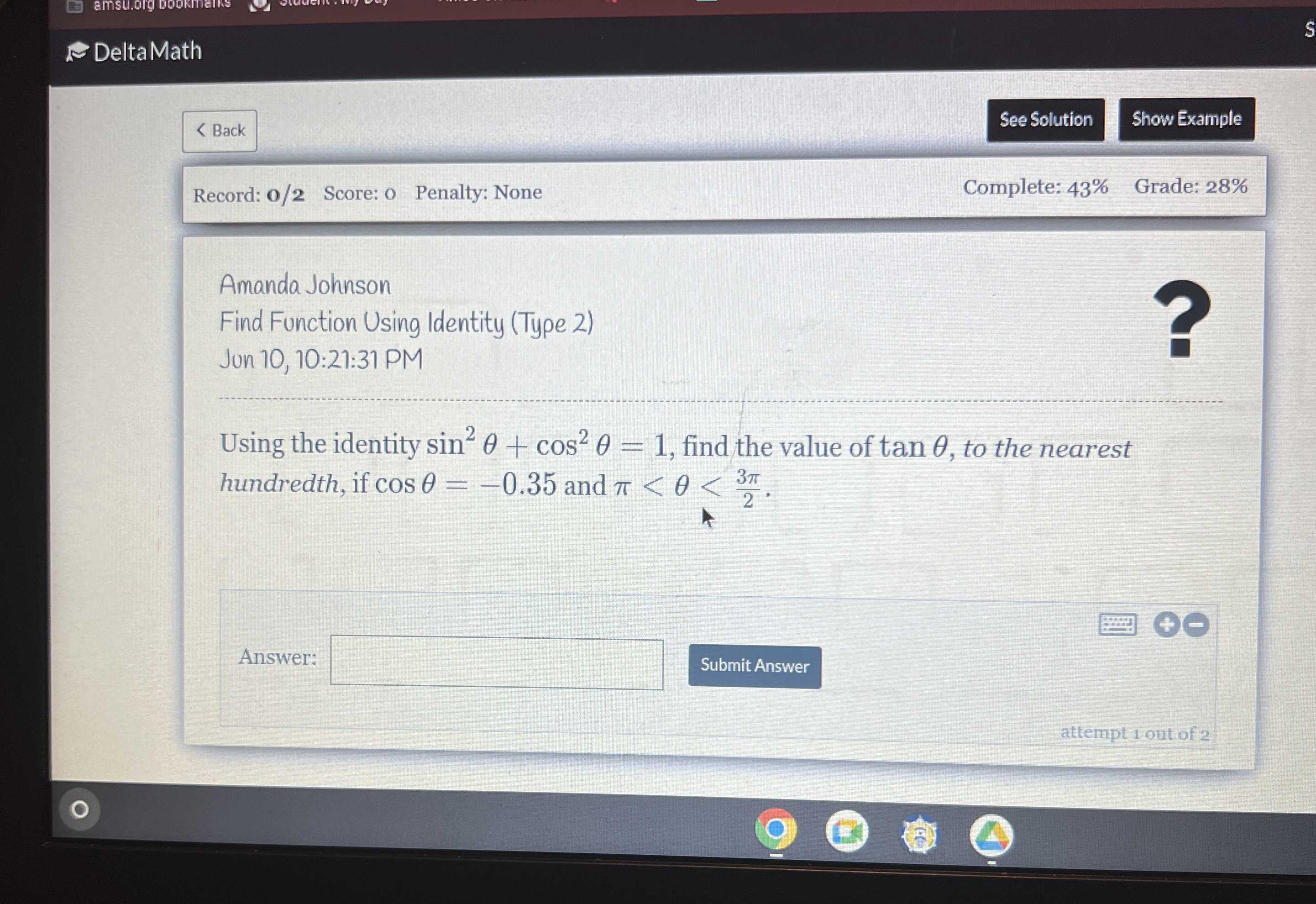### Still have math questions?Using the identity $$\sin ^ { 2 } \theta + \cos ^ { 2 } \theta = 1$$ , find the value of $$\tan \theta$$ , to the nearest hundredth, if $$\cos \theta = - 0.35$$ and $$\pi < \theta < \frac { 3 \pi } { 2 }$$ .